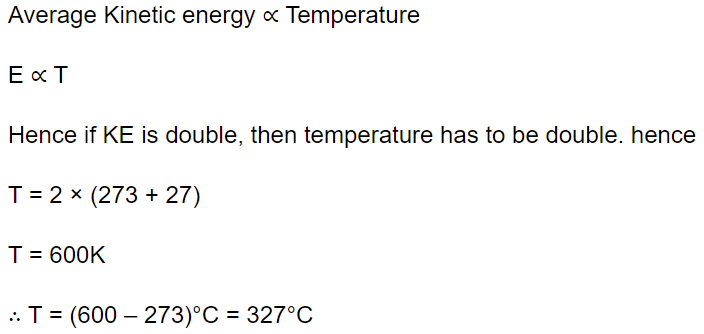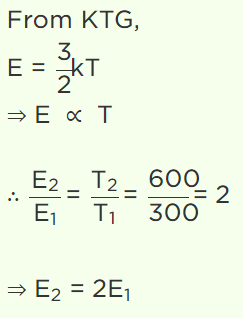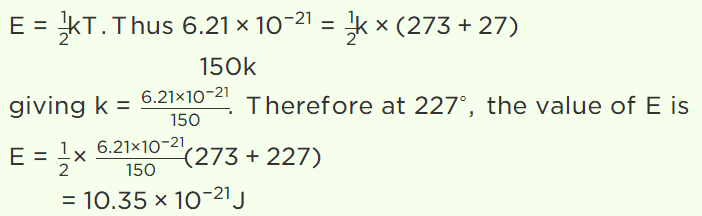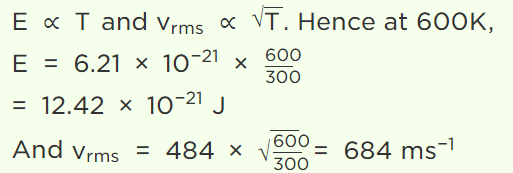## Kinetic Theory Questions and Answers Part-24

1. At what temperature is the kinetic energy of a gas molecule double that of its value of 27°C
a) 54°C
b) 300 K
c) 327°C
d) 108°C

Explanation:2. Read the given statements and decide which is/are correct on the basis of kinetic theory of gases
(I) Energy of one molecule at absolute temperature is zero
(II) r.m.s. speeds of different gases are same at same temperature
(III) For one gram of all ideal gas kinetic energy is same at same temperature
(IV) For one mole of all ideal gases mean kinetic energy is same at same temperature
a) All are correct
b) I and IV are correct
c) IV is correct
d) None of these

Explanation: None of these

3. The translational kinetic energy of gas molecule for one mole of the gas is equal to
a) $\frac{3}{2}RT$
b) $\frac{2}{3}RT$
c) $\frac{1}{2}RT$
d) $\frac{2}{3}KT$

Explanation: The translational kinetic energy of gas molecule for one mole of the gas is equal to $\frac{3}{2}RT$

4. At 27°C temperature, the kinetic energy of an ideal gas is E1 . If the temperature is increased to 327°C, then kinetic energy would be
a) $2E_{1}$
b) $\frac{1}{2}E_{1}$
c) $\sqrt{2}E_{1}$
d) $\frac{1}{\sqrt{2}}E_{1}$

Explanation:5. The average kinetic energy of a gas molecule at 27°C is $6.21\times 10^{-21}J$  . Its average kinetic energy at 227°C will be
a) $52.2\times 10^{-21}J$
b) $5.22\times 10^{-21}J$
c) $10.35\times 10^{-21}J$
d) $11.35\times 10^{-21}J$

Explanation:6. The average translational kinetic energy of $O_{2}$ (molar mass 32) molecules at a particular temperature is 0.048 eV. The translational kinetic energy of $N_{2}$ (molar mass 28) molecules in eV at the same temperature is
a) 0.0015
b) 0.003
c) 0.048
d) 0.768

Explanation: 0.048

7. The average translational energy and the r.m.s. speed of molecules in a sample of oxygen gas at 300 K are $6.21\times 10^{-21}J$   and 484 m/s respectively. The corresponding values at 600 K are nearly (assuming ideal gas behaviour)
a) $12.42\times 10^{-21}J$   ,968 m / s
b) $8.78\times 10^{-21}J$   ,684 m / s
c) $6.21\times 10^{-21}J$   ,968 m / s
d) $12.42\times 10^{-21}J$   ,684 m / s

Explanation:8. A polyatomic gas with n degrees of freedom has a mean energy per molecule given by
a) $\frac{nkT}{N}$
b) $\frac{nkT}{2N}$
c) $\frac{nkT}{2}$
d) $\frac{3kT}{2}$

Explanation: A polyatomic gas with n degrees of freedom has a mean energy per molecule given by $\frac{nkT}{2}$

9. At 0 K which of the following properties of a gas will be zero
a) Kinetic energy
b) Potential energy
c) Vibrational energy
d) Density